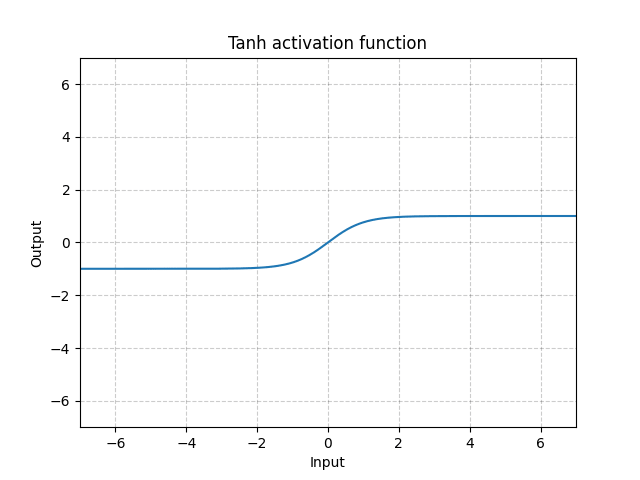/PyTorch

# Tanh

class torch.nn.Tanh [source]

Applies the element-wise function:

$\text{Tanh}(x) = \tanh(x) = \frac{\exp(x) - \exp(-x)} {\exp(x) + \exp(-x)}$
Shape:
• Input: $(N, *)$ where * means, any number of additional dimensions
• Output: $(N, *)$ , same shape as the inputExamples:

>>> m = nn.Tanh()
>>> input = torch.randn(2)
>>> output = m(input)


© 2019 Torch Contributors
Licensed under the 3-clause BSD License.
https://pytorch.org/docs/1.7.0/generated/torch.nn.Tanh.html